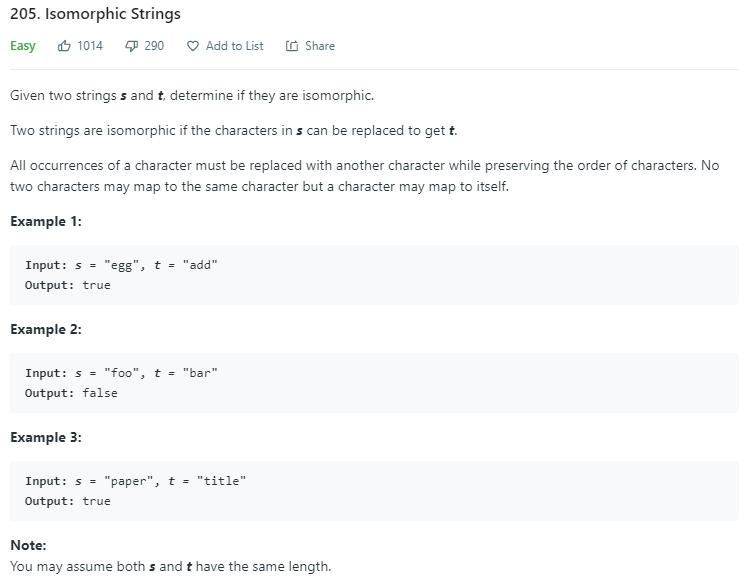# 题目描述（简单难度）# 解法一

egg 和 add 同构，就意味着下边的映射成立
e -> a
g -> d

a -> e
d -> g

foo 和 bar 不同构，原因就是映射不唯一
o -> a
o -> r



private boolean isIsomorphicHelper(String s, String t) {
int n = s.length();
HashMap<Character, Character> map = new HashMap<>();
for (int i = 0; i < n; i++) {
char c1 = s.charAt(i);
char c2 = s.charAt(i);
if (map.containsKey(c1)) {
if (map.get(c1) != c2) {
return false;
}
} else {
map.put(c1, c2);
}
}
return true;
}


public boolean isIsomorphic(String s, String t) {
return isIsomorphicHelper(s, t) && isIsomorphicHelper(t, s);

}

private boolean isIsomorphicHelper(String s, String t) {
int n = s.length();
HashMap<Character, Character> map = new HashMap<>();
for (int i = 0; i < n; i++) {
char c1 = s.charAt(i);
char c2 = t.charAt(i);
if (map.containsKey(c1)) {
if (map.get(c1) != c2) {
return false;
}
} else {
map.put(c1, c2);
}
}
return true;
}


# 解法二

将第一个出现的字母映射成 1，第二个出现的字母映射成 2

e -> 1
g -> 2

a -> 1
d -> 2

egg -> 122, add -> 122



public boolean isIsomorphic(String s, String t) {
return isIsomorphicHelper(s).equals(isIsomorphicHelper(t));
}

private String isIsomorphicHelper(String s) {
int[] map = new int;
int n = s.length();
StringBuilder sb = new StringBuilder();
int count = 1;
for (int i = 0; i < n; i++) {
char c = s.charAt(i);
//当前字母第一次出现,赋值
if (map[c] == 0) {
map[c] = count;
count++;
}
sb.append(map[c]);
}
return sb.toString();
}


public boolean isIsomorphic(String s, String t) {
return isIsomorphicHelper(s).equals(isIsomorphicHelper(t));
}

private String isIsomorphicHelper(String s) {
int[] map = new int;
int n = s.length();
StringBuilder sb = new StringBuilder();
for (int i = 0; i < n; i++) {
char c = s.charAt(i);
//当前字母第一次出现,赋值
if (map[c] == 0) {
map[c] = i + 1;
}
sb.append(map[c]);
}
return sb.toString();
}


对 abaddee 和 cdbccff

a b a d d e e
c d b c c f f
^

a -> 0
c -> 0

a -> 1
c -> 1

a b a d d e e
c d b c c f f
^

b -> 0
d -> 0

b -> 2
d -> 2

a b a d d e e
c d b c c f f
^

a -> 1 (之前被修改过)
b -> 0



public boolean isIsomorphic(String s, String t) {
int n = s.length();
int[] mapS = new int;
int[] mapT = new int;
for (int i = 0; i < n; i++) {
char c1 = s.charAt(i);
char c2 = t.charAt(i);
//当前的映射值是否相同
if (mapS[c1] != mapT[c2]) {
return false;
} else {
//是否已经修改过，修改过就不需要再处理
if (mapS[c1] == 0) {
mapS[c1] = i + 1;
mapT[c2] = i + 1;
}
}
}
return true;
}